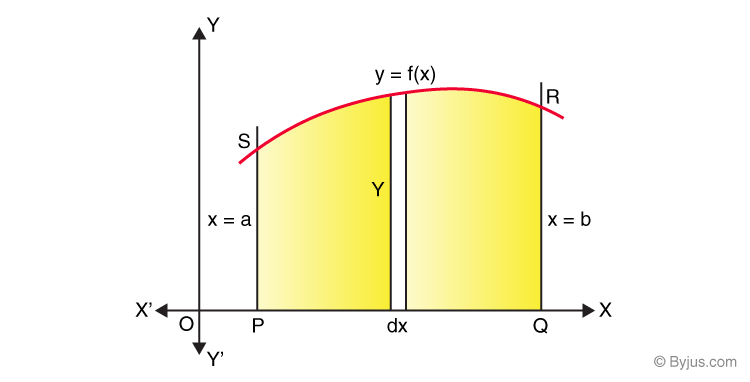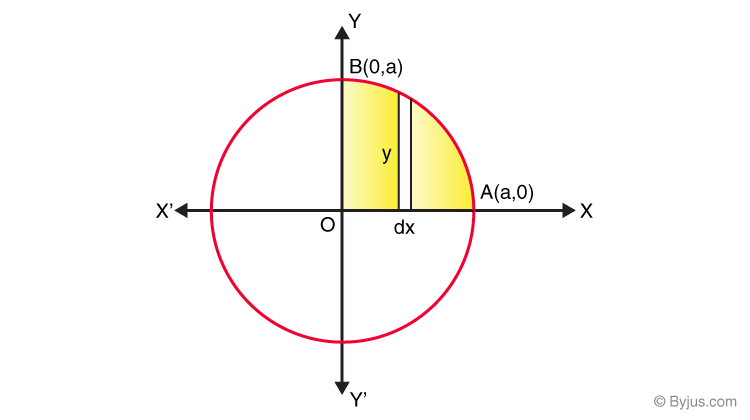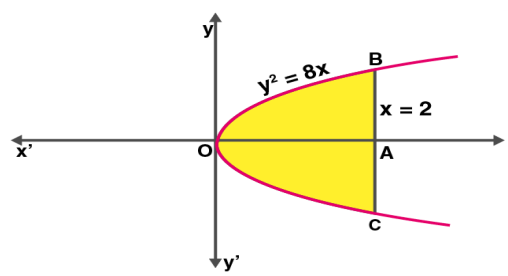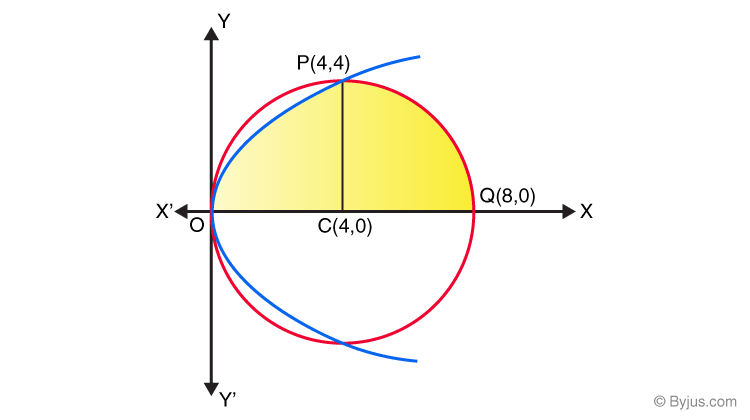# Application of Integrals for Class 12

From the previous classes, we are already aware of the various method of integration in Maths.  In the class 12 maths chapter 2, students will learn a specific application of integrals to find the area under simple curves, area between lines and arcs of standard forms of different curves such as circles, parabolas and ellipses. We shall also deal with finding the area bounded by these curves along with the combination of lines. In this article, let us look at the various application of integrals Class 12 Maths Chapter 8.

## Application of Integrals Class 12 Concepts

Chapter 8 – Application of Integrals class 12  CBSE covers the following concepts:

• Introduction
• Areas and Simple curves
• The area of a region bounded by a curve and a line
• The area between the two curves

From the previous classes, we are already aware of the various method of integration in maths.  In this article, let us look at the various application of integrals class 12 Maths.

One of the major application of integrals is in determining the area under the curves.

Consider a function y = f(x), then the area is given as$$\begin{array}{l}A = \int_{a}^{b} dA = \int_{a}^{b}y.dx = \int_{a}^{b}f(x)dx\end{array}$$

Consider the two curves having equation of f(x) and g(x), the area between the region a,b of the two curves is given as-

dA = f(x) – g(x)]dx, and the total area A can be taken as-

$$\begin{array}{l}A = \int_{a}^{b}[f(x) – g(x)]dx\end{array}$$

### Application of Integrals Examples

Example 1:

Determine the area enclosed by the circle x2 + y2 = a2

Solution:

Given, circle equation is x2 + y2 = a2From the given figure, we can say that the whole area enclosed by the given circle is as

= 4(Area of the region AOBA bounded by the curve, coordinates x=0 and x=a, and the x-axis)

As the circle is symmetric about both x-axis and y-axis, the equation can be written as

= 4 0a y dx (By taking the vertical strips) ….(1)

From the given circle equation, y can be written as

y = ±√(a2-x2)

As the region, AOBA lies in the first quadrant of the circle, we can take y as positive, so the value of y becomes √(a2-x2)

Now, substitute y = √(a2-x2) in equation (1), we get

= 4 0a √(a2-x2) dx

Integrate the above function, we get

= 4 [(x/2)√(a2-x2) +(a2/2)sin-1(x/a)]0 a

Now, substitute the upper and lower limit, we get

= 4[{(a/2)(0)+(a2/2)sin-1 1}-{0}]

= 4(a2/2)(π/2)

= πa2.

Hence, the area enclosed by the circle x2 +y2 =a2  is πa2.

Let’s have a look at the example to understand how to find the area of the region bounded by a curve and a line.

Example 2:

Find the area of the region bounded between the line x = 2 and the parabola y2 = 8x.

Solution:

Given equation of parabola is y2 = 8x.

Equation of line is x = 2.Here, y2 = 8x as a right handed parabola having its vertex at the origin and x = 2 is the line which is parallel to y-axis at x = 2 units distance

Similarly,

y2 = 8x has only even power of y and is symmetrical about x-axis.

So, the required area = Area of OAC + Area of OAB

= 2 (Area of OAB)

= 2 ∫02 y dx

Substituting the value of y, i.e. y2 = 8x and y = √(8x) = 2 √2 √x, we get;

= 2 ∫02 (2 √2 √x) dx

= 4√2 ∫02 (√x) dx

= 4√2 [x3/2/ (3/2)]02

By applying the limits,

= 4√2 {[23/2/ (3/2)] – 0}

= (8√2/3)  × 2√2

= (16 × √2 × √2)

= 32/3

Go through the example given below to learn how to find the area between two curves.

Example 3:

Determine the area which lies above the x-axis and included between the circle and parabola, where the circle equation is given as x2+y2 = 8x, and parabola equation is y2 = 4x.

Solution:

The circle equation x2+y2 = 8x can be written as (x-4)2+y2=16. Hence, the centre of the circle is (4, 0), and the radius is 4 units. The intersection of the circle with the parabola y2 = 4x is as follows:

Now, substitute y2 = 4x in the given circle equation,

x2+4x = 8x

x2– 4x = 0

On solving the above equation, we get

x=0 and x=4

Therefore, the point of intersection of the circle and the parabola above the x-axis is obtained as O(0,0) and P(4,4).Hence, from the above figure, the area of the region OPQCO included between these two curves above the x-axis is written as

= Area of OCPO + Area of PCQP

= 04 y dx + 48 y dx

= 2 04 √x dx + 48  √[42– (x-4)2]dx

Now take x-4 = t, then the above equation is written in the form

= 2 04 √x dx + 04  √[42– t2]dx …. (1)

Now, integrate the functions.

2 04 √x dx = (2)(⅔) (x3/2)04

2 04  √x dx = 32/3  …..(2)

04  √[42– t2]dx = [(t/2)(√[42-t2] + (½)(42)(sin-1(t/4)]04

04 √[42– t2]dx  = 4π …..(3)

Now, substitute (2) and (3) in (1), we get

= (32/3) +   4π

= (4/3) (8+3π)

Therefore, the area of the region that lies above the x-axis, and included between the circle and parabola is (4/3) (8+3π).